# 面试时写不出排序算法？看这篇就够了juejin.im/post/5cb6b8f551882532c334bcf2

## 冒泡排序

### 算法思想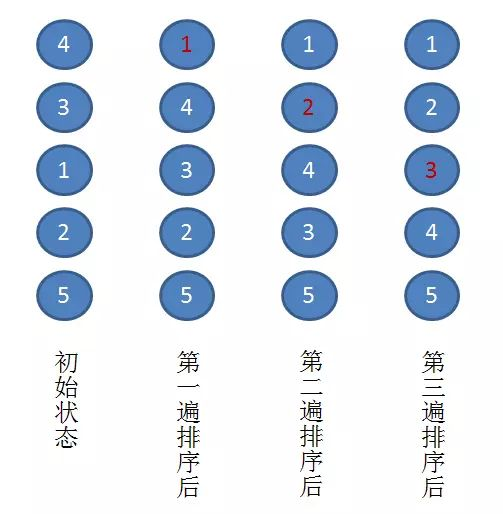• 第一趟排序：通过两两比较，找到第一小的数值 1 ，将其放在序列的第一位。

• 第二趟排序：通过两两比较，找到第二小的数值 2 ，将其放在序列的第二位。

• 第三趟排序：通过两两比较，找到第三小的数值 3 ，将其放在序列的第三位。

• 每趟排序过程中需要通过比较找到第 i 个小的元素。

• 所以，我们需要一个外部循环，从数组首端(下标 0) 开始，一直扫描到倒数第二个元素（即下标 N - 2) ，剩下最后一个元素，必然为最大。

• 所以，需要一个内部循环，从数组末端开始（下标 N - 1），扫描到 (下标 i + 1)。

public void bubbleSort(int[] list) {
int temp = 0; // 用来交换的临时数

// 要遍历的次数
for (int i = 0; i &lt; list.length - 1; i++) {
// 从后向前依次的比较相邻两个数的大小，遍历一次后，把数组中第i小的数放在第i个位置上
for (int j = list.length - 1; j &gt; i; j--) {
// 比较相邻的元素，如果前面的数大于后面的数，则交换
if (list[j - 1] &gt; list[j]) {
temp = list[j - 1];
list[j - 1] = list[j];
list[j] = temp;
}
}

System.out.format("第 %d 趟：\t", i);
printAll(list);
}
}


### 算法分析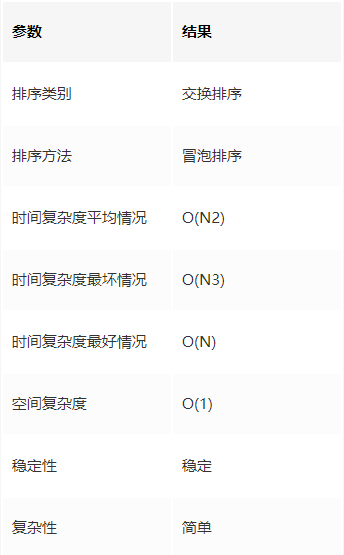### 时间复杂度

Cmax = N(N-1)/2 = O(N2)

### 优化

// 对 bubbleSort 的优化算法
public void bubbleSort_2(int[] list) {
int temp = 0; // 用来交换的临时数
boolean bChange = false; // 交换标志

// 要遍历的次数
for (int i = 0; i &lt; list.length - 1; i++) {
bChange = false;
// 从后向前依次的比较相邻两个数的大小，遍历一次后，把数组中第i小的数放在第i个位置上
for (int j = list.length - 1; j &gt; i; j--) {
// 比较相邻的元素，如果前面的数大于后面的数，则交换
if (list[j - 1] &gt; list[j]) {
temp = list[j - 1];
list[j - 1] = list[j];
list[j] = temp;
bChange = true;
}
}

// 如果标志为false，说明本轮遍历没有交换，已经是有序数列，可以结束排序
if (false == bChange)
break;

System.out.format("第 %d 趟：\t", i);
printAll(list);
}
}


### 示例代码

https://github.com/dunwu/algorithm-tutorial/blob/master/codes/algorithm/src/test/java/io/github/dunwu/algorithm/sort/SortStrategyTest.java

## 快速排序

### 算法思想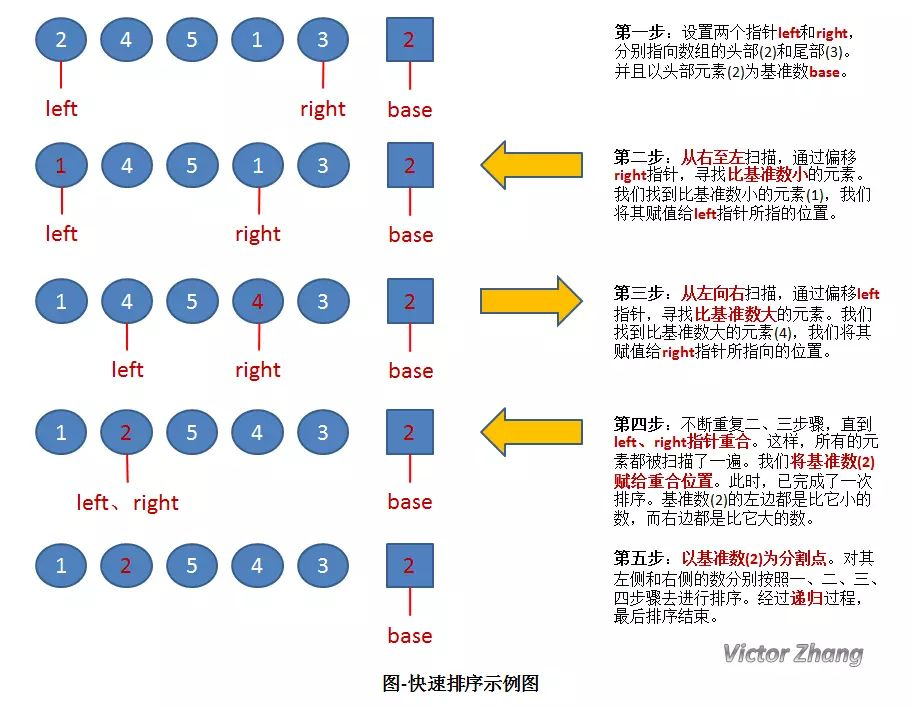1. 初始状态为一组无序的数组：2、4、5、1、3。

2. 经过以上操作步骤后，完成了第一次的排序，得到新的数组：1、2、5、4、3。

3. 新的数组中，以 2 为分割点，左边都是比 2 小的数，右边都是比 2 大的数。

4. 因为 2 已经在数组中找到了合适的位置，所以不用再动。

5. 2 左边的数组只有一个元素 1，所以显然不用再排序，位置也被确定。（注：这种情况时，left 指针和 right 指针显然是重合的。因此在代码中，我们可以通过设置判定条件 left 必须小于 right，如果不满足，则不用排序了）。

6. 而对于 2 右边的数组 5、4、3，设置 left 指向 5，right 指向 3，开始继续重复图中的一、二、三、四步骤，对新的数组进行排序。

public int division(int[] list, int left, int right) {
// 以最左边的数(left)为基准
int base = list[left];
while (left &lt; right) {
// 从序列右端开始，向左遍历，直到找到小于base的数
while (left &lt; right &amp;&amp; list[right] &gt;= base)
right--;
// 找到了比base小的元素，将这个元素放到最左边的位置
list[left] = list[right];

// 从序列左端开始，向右遍历，直到找到大于base的数
while (left &lt; right &amp;&amp; list[left] &lt;= base)
left++;
// 找到了比base大的元素，将这个元素放到最右边的位置
list[right] = list[left];
}

// 最后将base放到left位置。此时，left位置的左侧数值应该都比left小；
// 而left位置的右侧数值应该都比left大。
list[left] = base;
return left;
}

private void quickSort(int[] list, int left, int right) {

// 左下标一定小于右下标，否则就越界了
if (left &lt; right) {
// 对数组进行分割，取出下次分割的基准标号
int base = division(list, left, right);

System.out.format("base = %d:\t", list[base]);
printPart(list, left, right);

// 对“基准标号“左侧的一组数值进行递归的切割，以至于将这些数值完整的排序
quickSort(list, left, base - 1);

// 对“基准标号“右侧的一组数值进行递归的切割，以至于将这些数值完整的排序
quickSort(list, base + 1, right);
}
}


### 算法分析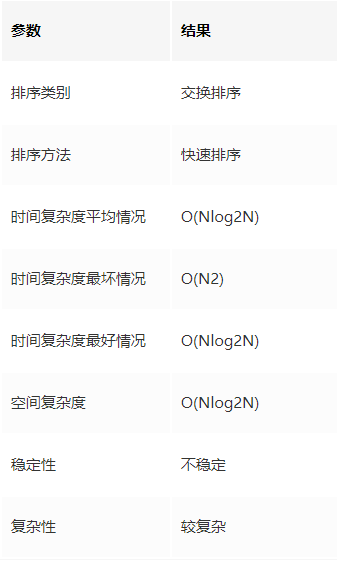### 示例代码

https://github.com/dunwu/algorithm-tutorial/blob/master/codes/algorithm/src/test/java/io/github/dunwu/algorithm/sort/SortStrategyTest.java

## 插入排序

### 算法思想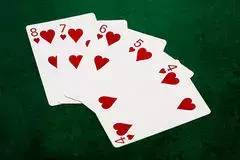• 先拿一张 5 在手里，

• 再摸到一张 4，比 5 小，插到 5 前面，

• 摸到一张 6，嗯，比 5 大，插到 5 后面，

• 摸到一张 8，比 6 大，插到 6 后面，

• 。。。

• 最后一看，我靠，凑到的居然是同花顺，这下牛逼大了。

• 我们先将这个序列中下标为 0 的元素视为元素个数为 1 的有序序列。

• 然后，我们要依次把 R1, R2, … , RN-1 插入到这个有序序列中。所以，我们需要一个外部循环，从下标 1 扫描到 N-1 。

• 接下来描述插入过程。假设这是要将 Ri 插入到前面有序的序列中。由前面所述，我们可知，插入 Ri 时，前 i-1 个数肯定已经是有序了。

public void insertSort(int[] list) {
// 打印第一个元素
System.out.format("i = %d:\t", 0);
printPart(list, 0, 0);

// 第1个数肯定是有序的，从第2个数开始遍历，依次插入有序序列
for (int i = 1; i &lt; list.length; i++) {
int j = 0;
int temp = list[i]; // 取出第i个数，和前i-1个数比较后，插入合适位置

// 因为前i-1个数都是从小到大的有序序列，所以只要当前比较的数(list[j])比temp大，就把这个数后移一位
for (j = i - 1; j &gt;= 0 &amp;&amp; temp &lt; list[j]; j--) {
list[j + 1] = list[j];
}
list[j + 1] = temp;

System.out.format("i = %d:\t", i);
printPart(list, 0, i);
}
}


### 算法分析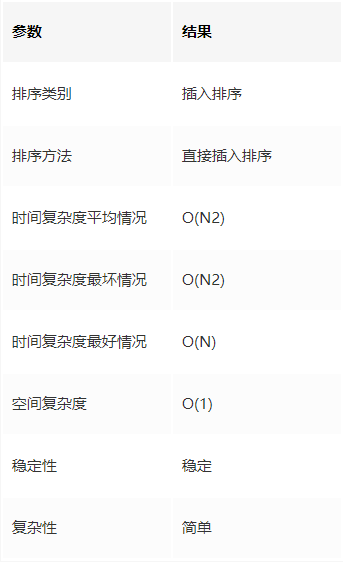### 算法稳定性

https://github.com/dunwu/algorithm-tutorial/blob/master/codes/algorithm/src/test/java/io/github/dunwu/algorithm/sort/SortStrategyTest.java

## 希尔排序

### 算法思想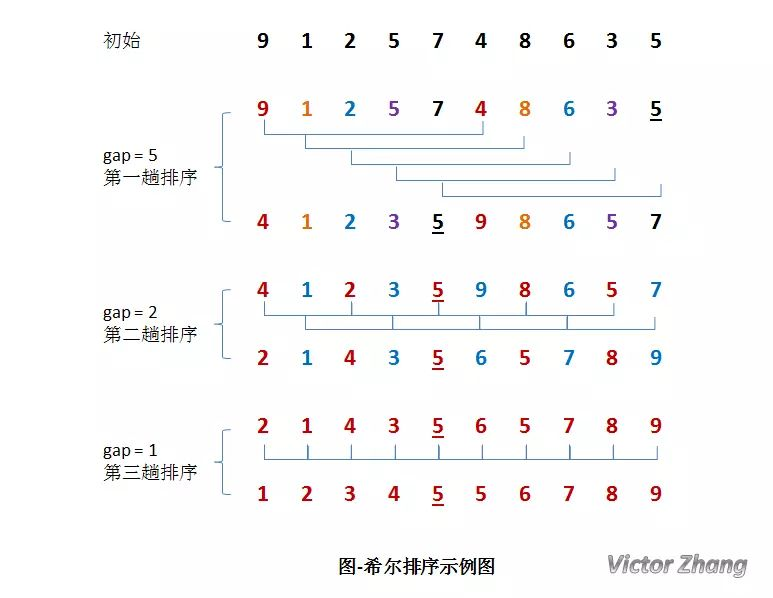• 接下来，按照直接插入排序的方法对每个组进行排序。

• 按照直接插入排序的方法对每个组进行排序。

• 按照直接插入排序的方法对每个组进行排序。此时，排序已经结束。

public void shellSort(int[] list) {
int gap = list.length / 2;

while (1 &lt;= gap) {
// 把距离为 gap 的元素编为一个组，扫描所有组
for (int i = gap; i &lt; list.length; i++) {
int j = 0;
int temp = list[i];

// 对距离为 gap 的元素组进行排序
for (j = i - gap; j &gt;= 0 &amp;&amp; temp &lt; list[j]; j = j - gap) {
list[j + gap] = list[j];
}
list[j + gap] = temp;
}

System.out.format("gap = %d:\t", gap);
printAll(list);
gap = gap / 2; // 减小增量
}
}


### 算法分析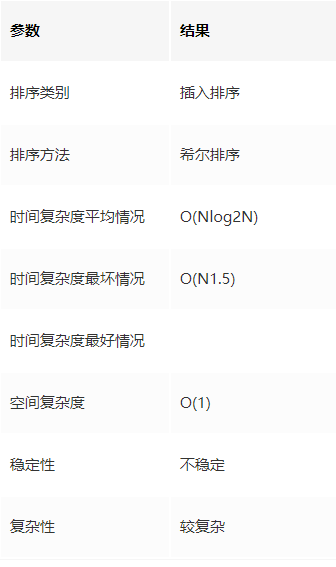### 时间复杂度

Donald Shell 最初建议步长选择为 N/2 并且对步长取半直到步长达到 1。虽然这样取可以比 O(N2)类的算法（插入排序）更好，但这样仍然有减少平均时间和最差时间的余地。可能希尔排序最重要的地方在于当用较小步长排序后，以前用的较大步长仍然是有序的。

### 直接插入排序和希尔排序的比较

• 直接插入排序是稳定的；而希尔排序是不稳定的。

• 直接插入排序更适合于原始记录基本有序的集合。

• 希尔排序的比较次数和移动次数都要比直接插入排序少，当 N 越大时，效果越明显。

• 在希尔排序中，增量序列 gap 的取法必须满足：**最后一个步长必须是 1 。**

• 直接插入排序也适用于链式存储结构；希尔排序不适用于链式结构。

https://github.com/dunwu/algorithm-tutorial/blob/master/codes/algorithm/src/test/java/io/github/dunwu/algorithm/sort/SortStrategyTest.java

## 简单选择排序

### 算法思想

• 从待排序序列中，找到关键字最小的元素；

• 如果最小元素不是待排序序列的第一个元素，将其和第一个元素互换；

• 从余下的 N - 1 个元素中，找出关键字最小的元素，重复 1、2 步，直到排序结束。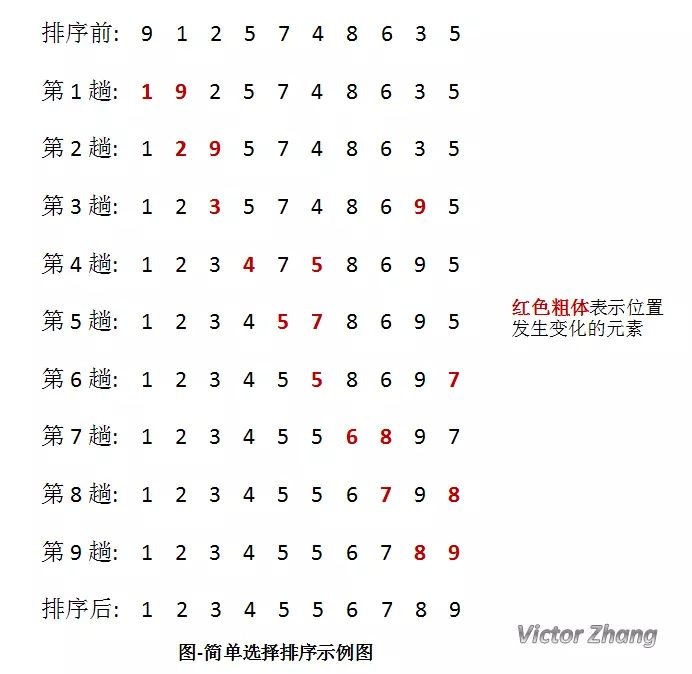### 算法分析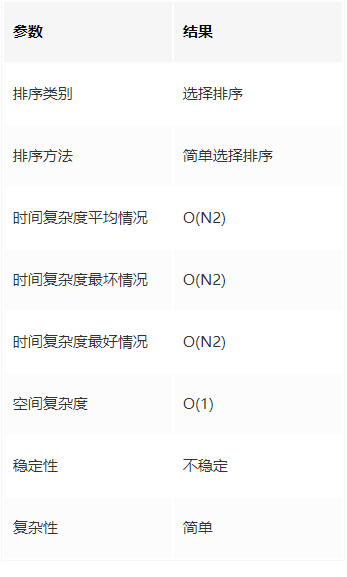### 空间复杂度

https://github.com/dunwu/algorithm-tutorial/blob/master/codes/algorithm/src/test/java/io/github/dunwu/algorithm/sort/SortStrategyTest.java

## 堆排序

### 要点

• Ri <= R2i+1 且 Ri <= R2i+2 （小根堆）

• Ri >= R2i+1 且 Ri >= R2i+2 （大根堆）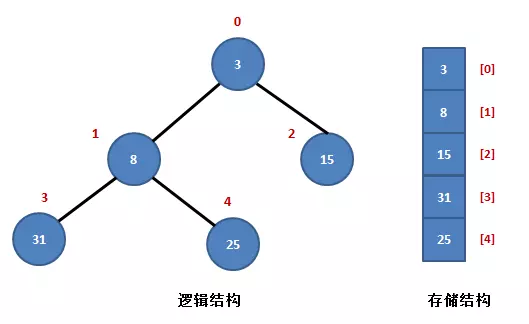• 它的左孩子结点是：R[2*i+1];

• 它的右孩子结点是：R[2*i+2];

• 它的父结点是：R[(i-1)/2];

• R[i] <= R[2*i+1] 且 R[i] <= R[2i+2]。

### 算法思想

• 首先，按堆的定义将数组 R[0..n]调整为堆（这个过程称为创建初始堆），交换 R和 R[n]；

• 然后，将 R[0..n-1]调整为堆，交换 R和 R[n-1]；

• 如此反复，直到交换了 R和 R为止。

• 根据初始数组去构造初始堆（构建一个完全二叉树，保证所有的父结点都比它的孩子结点数值大）。

• 每次交换第一个和最后一个元素，输出最后一个元素（最大值），然后把剩下元素重新调整为大根堆。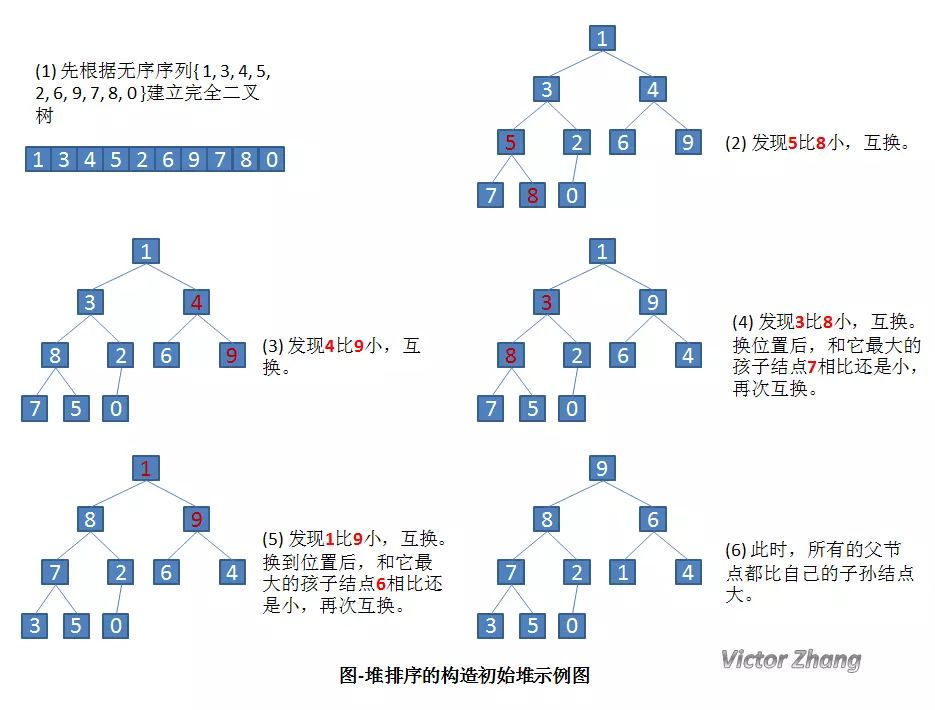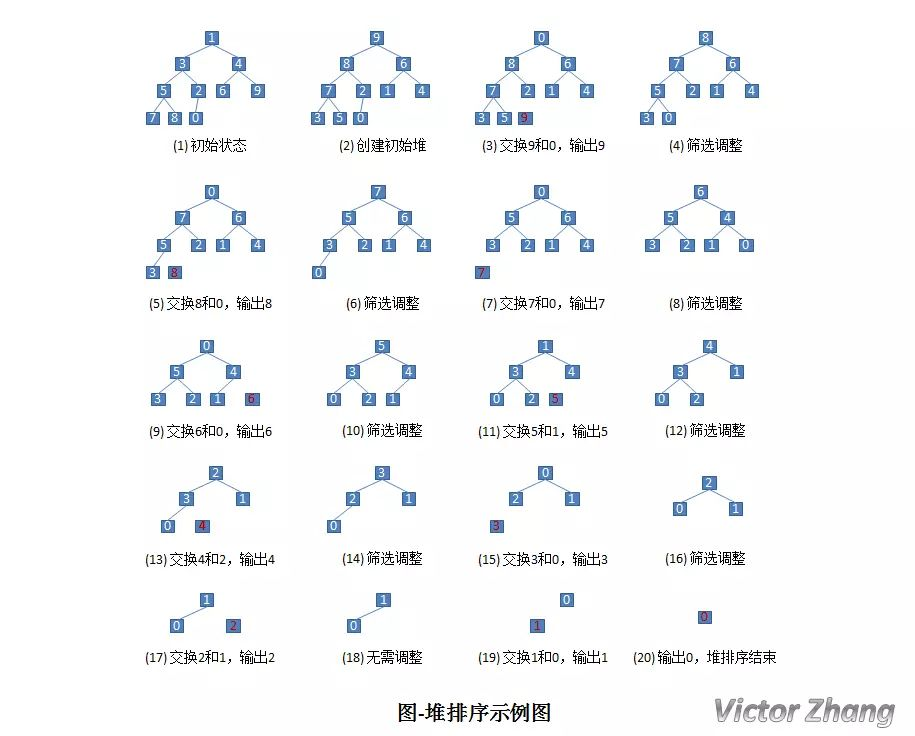public void HeapAdjust(int[] array, int parent, int length) {
int temp = array[parent]; // temp保存当前父节点
int child = 2 * parent + 1; // 先获得左孩子

while (child &lt; length) {
// 如果有右孩子结点，并且右孩子结点的值大于左孩子结点，则选取右孩子结点
if (child + 1 &lt; length &amp;&amp; array[child] &lt; array[child + 1]) {
child++;
}

// 如果父结点的值已经大于孩子结点的值，则直接结束
if (temp &gt;= array[child])
break;

// 把孩子结点的值赋给父结点
array[parent] = array[child];

// 选取孩子结点的左孩子结点,继续向下筛选
parent = child;
child = 2 * child + 1;
}

array[parent] = temp;
}

public void heapSort(int[] list) {
// 循环建立初始堆
for (int i = list.length / 2; i &gt;= 0; i--) {
}

// 进行n-1次循环，完成排序
for (int i = list.length - 1; i &gt; 0; i--) {
// 最后一个元素和第一元素进行交换
int temp = list[i];
list[i] = list;
list = temp;

// 筛选 R 结点，得到i-1个结点的堆
System.out.format("第 %d 趟: \t", list.length - i);
printPart(list, 0, list.length - 1);
}
}


### 算法分析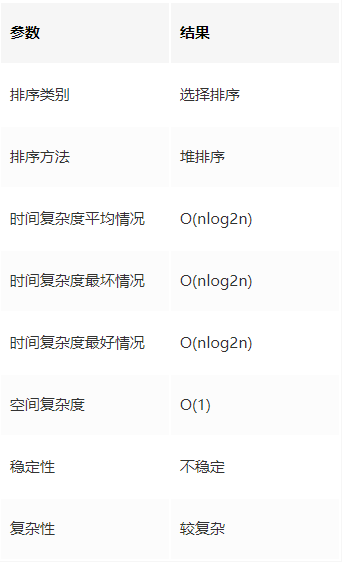### 示例代码

https://github.com/dunwu/algorithm-tutorial/blob/master/codes/algorithm/src/test/java/io/github/dunwu/algorithm/sort/SortStrategyTest.java

## 归并排序

### 算法思想

• “分解”——将序列每次折半划分。

• “合并”——将划分后的序列段两两合并后排序。

public void Merge(int[] array, int low, int mid, int high) {
int i = low; // i是第一段序列的下标
int j = mid + 1; // j是第二段序列的下标
int k = 0; // k是临时存放合并序列的下标
int[] array2 = new int[high - low + 1]; // array2是临时合并序列

// 扫描第一段和第二段序列，直到有一个扫描结束
while (i &lt;= mid &amp;&amp; j &lt;= high) {
// 判断第一段和第二段取出的数哪个更小，将其存入合并序列，并继续向下扫描
if (array[i] &lt;= array[j]) {
array2[k] = array[i];
i++;
k++;
} else {
array2[k] = array[j];
j++;
k++;
}
}

// 若第一段序列还没扫描完，将其全部复制到合并序列
while (i &lt;= mid) {
array2[k] = array[i];
i++;
k++;
}

// 若第二段序列还没扫描完，将其全部复制到合并序列
while (j &lt;= high) {
array2[k] = array[j];
j++;
k++;
}

// 将合并序列复制到原始序列中
for (k = 0, i = low; i &lt;= high; i++, k++) {
array[i] = array2[k];
}
}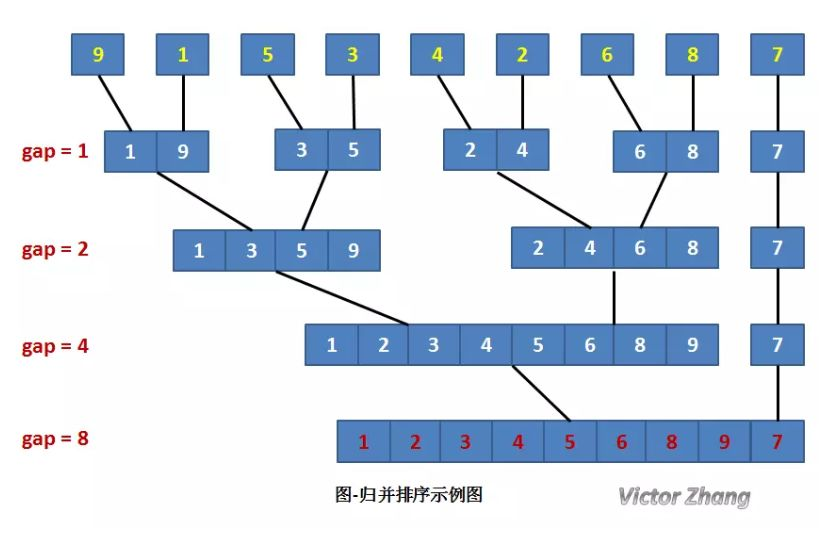public void MergePass(int[] array, int gap, int length) {
int i = 0;

// 归并gap长度的两个相邻子表
for (i = 0; i + 2 * gap - 1 &lt; length; i = i + 2 * gap) {
Merge(array, i, i + gap - 1, i + 2 * gap - 1);
}

// 余下两个子表，后者长度小于gap
if (i + gap - 1 &lt; length) {
Merge(array, i, i + gap - 1, length - 1);
}
}

public int[] sort(int[] list) {
for (int gap = 1; gap &lt; list.length; gap = 2 * gap) {
MergePass(list, gap, list.length);
System.out.print("gap = " + gap + ":\t");
this.printAll(list);
}
return list;
}


### 算法分析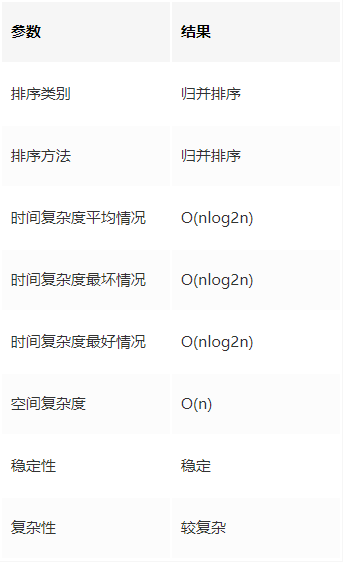### 归并排序和堆排序、快速排序的比较

• 若从空间复杂度来考虑：首选堆排序，其次是快速排序，最后是归并排序。

• 若从稳定性来考虑，应选取归并排序，因为堆排序和快速排序都是不稳定的。

• 若从平均情况下的排序速度考虑，应该选择快速排序。

https://github.com/dunwu/algorithm-tutorial/blob/master/codes/algorithm/src/test/java/io/github/dunwu/algorithm/sort/SortStrategyTest.java

## 基数排序

### 要点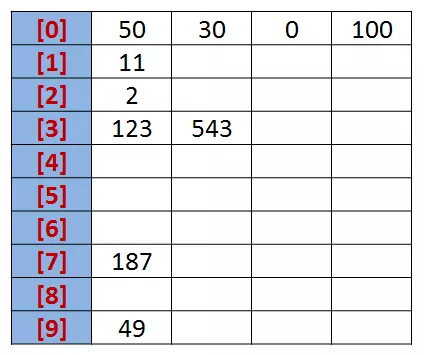### 算法分析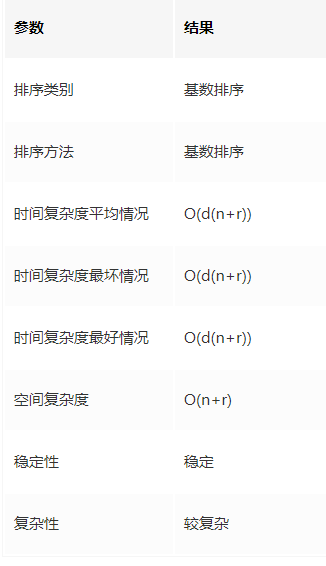### 示例代码

https://github.com/dunwu/algorithm-tutorial/blob/master/codes/algorithm/src/test/java/io/github/dunwu/algorithm/sort/SortStrategyTest.java推荐阅读

"2019，别去互联网大厂了！"